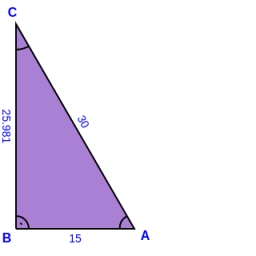# Calculate 46541

Calculate the perimeter and the area of a right triangle if a = 6 cm, b = 8 cm, c = 10 cm.

o =  24 cm
S =  24 cm2

### Step-by-step explanation:Did you find an error or inaccuracy? Feel free to write us. Thank you!

Tips for related online calculators

#### Grade of the word problem:

We encourage you to watch this tutorial video on this math problem: